Next: Comparison of EGO and Up: Output Files Previous: EGO Energy Summary Files

## Format of the flooding' matrix file flooding.lis

The file flooding.lis is used by EGO for performing rotation/translation correction and computation of the flooding forces. It contains:

• the number of flooding matrices to be used (currently mkflood supports only one matrix),
• the total number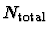of atoms within the system,
• the number N of selected atoms,
• a list ofselection flags (0:not selected, 1:selected) denoting which atom has been selected,
• the 3N averaged coordinates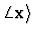of the selected atoms,
• the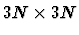elements of the (symmetric) flooding matrix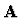, which determines the flooding potential,
• and (optionally) a list of the eigenvalues (eventually extrapolated) of the covariance matrix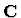(only for the human user).
The format of flooding.lis is:

[HEADER]
<nr_of_flooding_matrices>
<total number of atoms>
<number of selected atoms>
[USINGARRAY]
<selection flag of atom 1>
<selection flag of atom 2>
...
<selection flag of atom Ntotal>
[CENTER_OF_MASS]
<x-coordinate of 1st selected atom>
<y-coordinate of 1st selected atom>
<z-coordinate of 1st selected atom>
<x-coordinate of 2nd selected atom>
<y-coordinate of 2nd selected atom>
<z-coordinate of 2nd selected atom>
...
<x-coordinate of Nth selected atom>
<y-coordinate of Nth selected atom>
<z-coordinate of Nth selected atom>
[FLOODING_MATRIX]
<x1x1>
<x1y1>
<x1z1>
<x1x2>
<x1y2>
<x1z2>
...
<x1xN>
<x1yN>
<x1zN>
<y1x1>
<y1y1>
<y1z1>
...
<y1xN>
<y1yN>
<y1zN>
...
...
...
<zNxN>
<zNyN>
<zNzN>
[COVARIANCE EIGENVALUES]
<LAMBDA 1>  (smallest eigenvalue)
<LAMBDA 2>
...
<LAMBDA 3N> (largest eigenvalue)
<EOF>
`

Helmut Heller
2000-04-19